# Structural Description of X-LAM Members#

Cross-Laminated Timber(CLT or X-LAM) : A prefabricated engineered wood product consisting of not less than three layers of solid-sawn lumber or structural composite lumber where the adjacent layers are cross oriented and bonded with structural adhesive to form a solid wood element.  Hivatkozások a References fejezet listájár

Figure 1. Composition of X-LAM members

The steps of production of X-LAM members are the following:

• harvesting, lumber selection, logging, grouping, etc.
• creating lamellas(planks) by cutting to length
• creating single layer elements
• creating members(panels or billets) from the single layer elements
• quality control, machining, packaging, shipping, etc.

Materials used in X-LAM products:

• The timber used in the manufacturing of X-LAM is generally kiln dried C24 timber together with a limited amount of C16 timber. With a moisture content of approximately 12% within  ± 3% (∗), the density of the timber ranges from 470-590 Kg/m3 (dependent on the material).
• Approximately 0.6% of an X-LAM panel is made from adhesives, there- fore the glue content is very low in proportion to the product . The main wood adhesives are melamine-urea-formaldehyde (MUF), one- component polyurethane (PUR), phenol-resorcinol-formaldehyde (PRF) and emulsion polymer isocyanate (EPI).

Depending on the manufacturer, the covering layers may be aligned to either the longitudinal or the transversal panel direction, with planks being optionally edge-glued or breadthways-glued. The longitudinal planks are usually finger-jointed (see Fig. 2) and glued.

Figure 2. Orientations of finger-joints 

# Mechanical Description of X-LAM Members #

## Material Constitutive Equations #

In this section we introduce the stress-strain relations which are necessary for further investigation. For a deeper introduction see . Throughout the text we will use the Voight index notation, where the expression σij denotes a normal strain if i = j and a shar strain otherwise: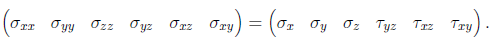1.

The notation of engineering strains with the same concept: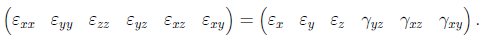2.

If the stress components are assumed to be linear functions of the strain components and we assume that the reference configuration is stress free, then the constitutive equations in contracted notation are: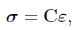3.

Where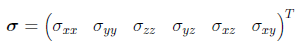4.

is the vector of stress components,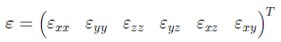5.

is the vector of engineering strain components, and C is the matrix of elastic material constants (elasticity matrix):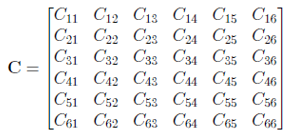6.

The relationship of equation (3) is assumed to be invertible, thus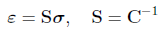7.

where S is the compliance matrix.

A reasonable assumption is to model timber as an orthotropic material, and rewrite Hook’s law in a coordinate system aligned to its anatomic structure. If we formulate the compliance matrix S in an orthogonal coordinate system {x1, x2, x3} such that x1, x2 and x3 are aligned to the longitudinal, radial and tangential directions, equations  and  can be written as: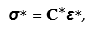8.9.

with the vectors of stress and strain in the material coordinate system:

 (10)

and

 (11)
 (a) Material coordinate system  (b) Layering scheme Figure 3. Lamination scheme of X-LAM members

The compliance matrix S∗ is characterized by the following 9 independent engineering constants:

 (12)

Coordinate Transformation

The equations so far were written with respect to the principal material coordinate system of the actual lamina (subscript m), which in most of the situations does not coincide with the problem coordinate system. Furthermore, a laminate in general case is composed of a set of laminas with different orientations (θ, see Figure 3a) of their principal material coordinate systems. Therefore there is a need to transform the relations among stresses and strains for each layer into the problem coordinate system.

The stresses transform according to:

 (13)

where

T is the transformation matrix.

Using the engineering strains introduces some awkwardness into the trans- formation of strain components, some of which can be reduced by using the so-called Reuter’s matrix R, hence

 (14)

Finally, the material coefficients transform according to:

 (15)

where

C is the elasticity tensor with respect to the problem coordinate system;

C∗ is the elasticity tensor with respect to the material coordinate system.

• ## Relations Among Material Properties#

According to section 2.1, for the proper definition of the material constitutive equations we need 9 independent engineering constants. Since the brochures provided by the X-LAM manufacturers usually publish less than that, we need other sources of information. We make use of the fact that X-LAM products are made from softwood, which reportedly share some relations among their stiffness parameters. These are based on the tabular data containing all of the 9 material constants of several softwood species native in Europe, North America and Russia provided by Hearmon , Bodig and Goodman  and Askenazi , and the related work of other researchers  .

• ## Member Constitutive Equations#

Current design models for X-LAM mainly emerged from the long tradition of using only one-dimensional elements in wood constructions, accordingly they rely on beam theoretical considerations to a great extent. Some of the most popular ones based on the literature review of Stürzenbecher et al. :

• Mechanically jointed beam theory (γ-method)

Introduced by Möhler , the crossing layers are considered as shear compliant connections instead of individual layers by introducing a correction factor to the bending stiffness. An important limitation of the method is that it only allows for the design of simply supported beams under sinusoidal loading.

• Shear analogy method

Introduced by Kreuzinger , the method is based on the separation of the two parts of overall bending stiffness of members: the individual stiffnesses of the lamellae and the stiffness increments by their joint action. These shares of bending stiffness and the shear stiffness of the individual layers are assigned to two beams showing coupled displacements. The method overcomes the limitations of the γ-method and compliant joints can also be easily considered.

• Timoshenko beam theory

The theoretically most straightforward from the beam approaches is the Timoshenko beam theory , recommended by Schickhofer et al.  for the structural design of X-LAM. It is reported by Bajczerová , that the method produces almost the same stiffness as the γ-method.

The so far introduced methods all suffer from the same deficiency as they account for a beam-like behaviour, and so neglect two-dimensional stress transfer. Furthermore, since these models do not provide the flexibility of defining an arbitrary 2-D domain and boundary conditions, they are simply inapplicable in a commercial finite element software such as AxisVM, and the application of a 2-D shell model is inevitable.

In the field of mechanics of laminated composites a multiplicity of plate theories were derived, where Equivalent Single Layer Methods (ESLM) show the computational convenience that the number of independent variables does not depend on the number of layers. In these, a laminated plate is considered as a statically equivalent single layer having complex constitutive behavior. On the contrary, Layer-Wise Methods (LWM) exhibit the same number of independent variables for each layer, and thus, show thoroughly increasing computational costs with increasing number of layers.

The Mindlin plate theory, or as it is often called, the first-order shear deformation plate theory (FSDT) proposed in 1951 by Raymond Mindlin  is a generalization of the Timoshenko beam theory for 2-D applications, and is already the main tool for the analysis of thick plates. The suitability of FSDT amongst other theories to reproduce deformations and stresses of point loaded CLT plates was examined by Czaderski et al. , and except from the 3D FE calculations and the Mindlin plate theory in case of deformations, considerable deviations of the computed results from corresponding experimental values were reported. The suitable stiffness representation of laminated plates by the Mindlin theory is also reported in . Based on comparisons with the exact analytical solutions of Pagano , it is also concluded by Stürzenbecher et al. , that although more advanced plate theories are capable of producing more accurate results even directly, the FSDT delivers an appropriate shear stress distribution, on the indirect cost that it has to be back-calculated from in-plane stresses by integration of the infinitesimal equilibrium equations.

Due to its reportedly suitable modeling capabilities for the case of laminated plates and keeping in mind it’s great integrability to a commercial code, the development of an equivalent single layer method is to be introduced in the next section on the basis of the Mindlin plate theory.

• ### Mindlin Plate Theory for Laminated Composite Shells#

Retaining the assumption of linearity of displacements but discarding the normal hypothesis of the so-called Classical Plate Theory (CPT), commonly known as Kirchhoff Plate Theory  for homogeneous plates, leads to the  Mindlin plate theory. The resulting strain field reads as:

 (16)

where

ε0 is the vector of engineering strains measured on the centerplane *

z is the shortest distance of the material point from the centerplane;

κ is the vector of curvatures.

We note that the actual definition of curvatures depends on the arbitrary **

sign conventions of the kinematic variables.

*the collection of points that satisfy 𝑥 = 0

**with the clause, that the curvatures must be energy consistent with the moments lábjegyzet

The lamination scheme illustrated on Figure 10, the internal forces and moments of a general flat shell are defined as:

 (17) (18) (19)

or in a more compact form

 (20)
• ### Shear Correction#

The displacement formulation of the Mindlin-Reissner theory offers a constant shear stress distribution through the thickness. Since this is not a realistic distribution, we apply correction factors kx and ky to have an energy consistent model.

The calculated shear correction factors for a typical X-LAM member are about 1/4 of that of a homogeneous rectangular cross section, which is 5/6. The calculation procedure is confirmed in .

We only mention that the shear correction factors could be calculated on the basis of a plate theory, but it leads to a nonlinear problem since the resulting factors are dependent on the internal force distribution within the domain .

• # Design of X-LAM Members#

EC5 requires that actions are assigned to one of the load duration classes of Table 1.

 Load-duration class Order of accumulated duration of characteristic load Permanent more than 10 years Long-term 6 months – 10 years Medium-term 1 week – 6 months Short-term less than one week Instantaneous

• ## Service classes#

Structures shall be assigned to one of the service classes given below:

• Service class 1 (SC1) is characterized by a moisture content in the materials corresponding to a temperature of 20 °C and the relative humidity of the surrounding air only exceeding 65 % for a few weeks per year.
• Service class 2 (SC2) is characterized by a moisture content in the materials corresponding to a temperature of 20 °C and the relative humidity of the surrounding air only exceeding 85 % for a few weeks per year.

• ## Modification Factors of Strength and Stiffness#

Effect of load-duration and moisture content on strength

The effect is taken into account by the modification factor kmod. The value of the parameter is selected on the basis of the relevant service class and load- duration class according to EC5.

Until there is no standardized agreement over the assignment of kmod, the values proposed in  are suggested, according to Table 2.

 Service class Load duration class Permanent Long- term Medium- term Short- term Instantaneous SC1 0.6 0.7 0.8 0.9 1.10 SC2 0.6 0.7 0.8 0.9 1.10

Table 2. Values of kmod for X-LAM

Effect of moisture content on deformation

The effect is taken into account by the modification factor kdef selected for a given service class according to Table 3. The values are based on the proposed values of  and  for service classes 1 and 2, and in the absence of approval, the value defined for plywood in EC5 for service class 3.

 SC1 SC2 SC3 0.8 1.0 2.5

Table 3. Values of kdef for X-LAM

Effect of member size on strength

For timber products, usually a modification factor kh is used to increase the characteristic strength values based on the cross sectional dimensions of the member and the dimensions of the reference specimen. Until there is no standardized agreement over the calculation of this parameter, a value of kh = 1.0 is used.

System strength

When several equally spaced similar members, components or assemblies are laterally connected by a continuous load distribution system, the member strength properties may be multiplied by a system strength factor ksys. The increase in strength only applies with parallel stressing of several boards, like with normal and bending stresses, however, not if only one board is subject to rolling shear (EN 1995-1-1, Section 6.6).

According to EC5, for glued or prestressed laminated timber decks or floors the value of ksys should be given by:

 (21)

where

n is the number of loaded laminations.

The application of the parameter can be explained by the fact, that the conventional design methodology of EC5 relies on a beam model, which neglects the Poisson effect.

Effect of cracks

According to EC5, for the verification of shear resistance of members in bending, the influence of cracks should be taken into account using the crack factor kcr.

The recommended values for kcr are given as

kcr = 0.67 for solid timber;

kcr = 0.67 for glued laminated timber;

kcr = 1.0 for other wood-based products in accordance with EN 13986 and EN 14374.

Furthermore, it is noted in  that using the crack factor is not necessary, since cross-laminated timber is a two-dimensional element with an interlocked build-up and possible cracks are assumed to be considered via the product approvals.

Effect of shape of cross section The effect on strength arising from the cross-sectional shape of beam members is taken into account by applying a factor kshape to the design shear strength. Since the concept of the parameter assumes the application of a beam model, a value of kshape = 1.0 is used here.

Effect of finger joints According to , the bending strength must be reduced by 30% for universally finger-jointed CLT elements. The reduction is taken into consideration via the factor kfin. When applied, the finger joints are assumed to only join longitudinal plank.

Partial factor for material properties The partial safety factor γM on the resistance side may be determined nationally and must be observed in any case.

If not defined nationally, the value of the parameter is taken as γM = 1.25 according to .

• ## Design Value of a Material Property#

The calculation of the design value of a material property χ according to EC5 is summarized in Table 4.

 Analysis type SLS ULS First order, linearly elastic Second order, linearly elastic Vibration

1 In the absence of data from previous calculation

φ2 = 1.0 is conservative.

Table 4. Calculation of stiffness parameters

The Poisson’s ratios should be used with their provided values in chapter 2.2.

• ## Resistance Properties of Timber#

As occurs for other engineered wood products, such as laminated veneer lumber (LVL) and glued-laminated timber (glulam) beams, the mechanical properties of CLT are manufacturer dependent. Commonly, mechanical properties are provided by each manufacturer on a proprietary basis, therefore consultation with each manufacture is essential during the design process. Table 5 presents strength values of X-LAM according to EN 338 and other relevant sources  :

 Bending strength parallel to grain 1 fm,0,k 24 Bending strength perpendicular to grain 2 fm,90,k 0.5 Tension strength parallel to grain ft,0,k 14 Tension strength perpendicular to grain ft,90,k 0.5 Compression strength parallel to grain fc,0,k 21 Compression strength perpendicular to grain fc,90,k 2.5 Shear strength fv,k 2.5 Rolling shear strength fr,k 1 Torsional shear strength fT,k 2.5

1 Denoted as fm,k in EC5.

2 Not defined in the current standards.

Table 5. Characteristic resistance values of C24 timber in N/mm2

according to EN 338

Notes:

• For universally finger-jointed CLT elements, the bending strength must be reduced.
• Shape and processing of the board layers decisively influence the rolling shear strength. Therefore here, in particular, reference is made to the product  approval.
If the boards are edge-glued of if, with missing edge gluing, a minimum width to thickness ratio of 4:1 is complied with, then according to EN 16351:2013.

For universally finger-jointed cross laminated timber elements, the flexural strength for stress upon use as a panel must be reduced.

• ## Design Value of a Resistance Property#

The design value χd of a resistance property shall be calculated as:

 (22)

where

χk is the characteristic value of the resistance property;

γM is the partial factor for the resistance property;

kmod is a modification factor taking into account the effect of the duration of load and moisture content. According to the previous sections, the elements of Table 5 are calculated

as:

ft,0,d = ft,0,k · kmod · ksysM ft,90,d = ft,90,k · kmod · ksysM fc,0,d = fc,0,k · kmodM

fc,90,d = fc,90,k · kmodM fv,d = fv,k · kmodM

fr,d = fr,k · kmodM

fm,0,d = fm,0,k · kmod · ksys · kf inM fm,90,d = ft,90,k · kmod · ksysM

• ## Design for Ultimate Limit States#

In this section we list the limit states to be checked according to EN 1995-1-1 , DIN 1052:2004-8  and various research papers    .

AxisVM performs the following checks only. All the other checks specified in the design code for supports, joints, etc. has to be completed by the user.

Combined bending and axial tension parallel to grain direction

 (23)

where

σt,0,d is the design tensile stress along the grain;

ft,0,d is the design tensile strength along the grain;

σm,0,d is the design bending stress along the grain;

fm,0,d is the design bending strength along the grain.

Combined bending and axial tension perpendicular to grain direc tion

 (24)

where

σt,90,d is the design tensile stress perpendicular to grain direction;

ft,90,d is the design tensile strength perpendicular to grain direction;

σm,90,d is the design bending stress perpendicular to grain direction;

fm,90,d is the design bending strength perpendicular to grain direction.

Combined bending and axial compression parallel to grain direction

 (25)

Where

σc,0,d is the design compressive stress along the grain;

fc,0,d is the design compressive strength along the grain;

σm,0,d is the design bending stress along the grain;

fm,0,d is the design bending strength along the grain.

Combined bending and axial compression perpendicular to grain direction

 (26)

Where

σc,90,d is the design compressive stress perpendicular to grain direction;

fc,90,d is the design compressive strength perpendicular to grain direction;

kc,90 is a factor taking into account the load configuration, the possibility of splitting and the degree of compressive deformation;

σm,90,d is the design bending stress perpendicular to grain direction;

fm,90,d is the design bending strength perpendicular to grain direction.

In the absence of a standardized value, applying kc,90 = 1.0 is on the safe side.

Combined shear and torsion

 (27)

Where

τT,d is the design torsional stress;

τd is the design shear stress;

τr,d is the design rolling shear stress;

fv,d is the design shear strength.

Combined rolling shear and axial tension perpendicular to grain direction

 (28)

Where
σt,90,d is the design tensile stress perpendicular to grain direction;
ft,90,d is the design tensile strength perpendicular to grain direction;

τr,d is the design rolling shear stress;

fr,d is the design rolling shear strength.

Combined rolling shear and axial compression perpendicular to grain direction

 (29)

Where

σc,90,d is the design compressive stress perpendicular to grain direction;

fc,90,d is the design compressive strength perpendicular to grain direction;

τr,d is the design rolling shear stress;

fr,d is the design rolling shear strength.

• ## Implementation in AxisVM#

• ### Definition#

Upon defining a domain and selecting ’XLAM’ from the list of possible domain types, a dedicated material is attached to the domain automatically with predefined strength and stiffness parameters introduced in sections 2.2 and 3.5

Figure 4. Material properties in AxisVM

X-LAM products can be selected from the XLAM library, where the orientation of the individual layers are with reference to the top layer grain direction (see  Figure 5), which is to be set for the domain along with the service class.

When defining a domain, the user can decide on the application of strength modification factors ksys and kfin described in section 3.5, by clicking the corresponding checkboxes (unchecked by default).

Figure 5. Material properties in AxisVM

Figure 6. A possible XLAM domain definition in AxisVM

• ### Results#

The stresses are available in the usual graphical representation styles (diagram, Isolines, Isosurfaces 2D, Isosurfaces 3D). As usual in timber design, the stresses are separated according to the source of membrane or plate effect. Stress components originated from bending are stored with their maximum and minimum values along the thickness direction. From the two, the one corresponding to a point with positive vertical coordinate will be denoted as ’top’ (e.g. Sxx m T), the other one with negative vertical coordinate as ’bottom’ (e.g. Sxx m B). The stress components caused by normal and shear forces are represented by the value through the thickness having the largest value in absolute sense. The representative stresses at a point of an arbitrary internal force scenario are marked with black dots on Figure 7.

Clicking on a node of a surface element draws the stress distribution along the thickness. If envelop diagrams are to be plotted, the load cases causing extreme values in the stress component being active will be highlighted with red(maximum) and blue(minimum), see Figure 8.

Figure 7. X-LAM stress distribution in AxisVM

The limit states of section 3.7 are grouped into 4 utilization categories as:

• M-N-0 utilization : equations (23) and (25)
• M-N-90 utilization : equations (24) and (26)
• V-T utilization : equation (27)
• Vr-N utilization : equations (28) and (29)

Figure 8. Stress distribution diagrams at a node, with sxy,m being the active  component.

• ### Tips and Tricks #

• The calculation and design of X-LAM members is currently not covered in the relevant standards. Always look up the brochures and ETA § documents of the manufacturers and check the definition of X-LAM   members.
• The resistance value ft,90,k for softwood is usually much lower than ft,0,k. If a point is under a biaxial state of stress, this usually results in high utilization value for component ’M-N-90’. Under these circumstances cracks may appear along the grains in the tension zone. To circumvent this failure is up to the designer.
• The European Technical Assessment (ETA) is an alternative for construction products not covered by a harmonised standard. It is a document providing information on their performance assessment.

• ## Verification Example 1#

Calculate the stresses and utilization of a simple example illustrated on Figure 9. The calculations are carried out with 4 significant digits and units understood in [kN ] and [cm].

Figure 9. Setup of example 1.

The parameters are the following:

name of the product : KLH 3(60)s TL

length : l = 600.0

width : b = 1.0

mean major modulus of elasticity : E0,mean = 1100

mean minor modulus of elasticity : E90,mean = 55.00

Top layer grain direction : x

thicknesses : (2, 2, 2)

thickness : t = 6.000

orientations : (ǁ, , ǁ)

service class : 1

distributed surface load value : p = 1E − 4

With hand calculations:

kdef = 0.8000

bending rigidity : EI = 19100.

maximum weighted statical moment : Smax = S(0) = 4427

 maximum bending moment at midspan: maximum shear force at the supports: maximum normal stress: maximum shear (rolling shear) stress: maximum deflection:

kmod = 0.6000

kfin = 1.000

γM = 1.250

 design bending strength: utilization of bending strength: 0.6748 design rolling shear strength: utilization of shear strength: 0.1448

Results with AxisVM (with ν = 0):

 (a) σxx distribution at midspan (b) σx𝑥 distribution at the right support Figure 10. Stress distributions of Example 1

Figure 11. Plot of ’Sxx m T’ of Example 1 in AxisVM

Figure 12. Plot of ’Sxz max’ of Example 1 in AxisVM

Figure 13. Deflection plot of Example 1

Figure 14. M-N-0 utilization plot of Example 1

Figure 15. Vr-N utilization plot of Example 1

• ## Verification Example 2#

In this example we illustrate the stiffness calculation of an X-LAM shell illustrated on Figure 16. The calculations are carried out with 4 significant   digits and units understood in [kN ] and [cm].

Figure 16. Setup of Example 2

The parameters are the following:

name of the product : Binderholz 7(260) 125

mean major modulus of elasticity : E0,mean = 1100

mean minor modulus of elasticity : E90,mean = 55.00

mean shear modulus: Gmean = 69.00

mean rolling shear modulus: Gr,mean = 6.900

Top layer grain direction : x

thicknesses : (6, 2, 4, 2, 4, 2, 6)

orientations : (ǁ, , ǁ, , ǁ, , ǁ)

service class : 1

distributed load value : p = 1E − 4

The introduced calculation is verified with ANSYS, using element type SHELL281 with multilayer capabilities.

Figure 17. Displacement plot of Example 2 in ANSYS with an element size of 25 cm

Figure 18. Displacement plot of Example 2 in AxisVM with an element size of 25 cm

# References#

1. E. Karacabeyli, B. Douglas, CLT Handbook : cross-laminated timber, Pointe-Claire, Quebec : FPInnovations, 2013 (2013).
2. X-lam allaince technical issue 1, Tech. rep.
3. R. W. Jokerst, Finger-jointed wood products, Tech. Rep. FPL-RP-382, Forest Products Laboratory (apr 1981).
4. R. Jones, Mechanics Of Composite Materials, Materials Science and Engineering Series, Taylor & Francis, 1998 (1998).
5. J. Reddy, Mechanics of Laminated Composite Plates and Shells: Theory and Analysis, Second Edition, CRC Press, 2004 (2004).
6. R. F. S. Hearmon, The Elasticity of Wood and Plywood, Forest products research, H.M. Stationery Office, 1948 (1948).
7. J. Bodig, J. Goodman, Prediction of elastic parameters for wood, Wood science 5(4) (1973) 249 – 264 (1973).
8. E. K. Askenazi, Anizotropia dreveszinü i drevesznüh materialov, Lesznaja Promüslennoszty, Moscow, 1978 (1978).
1. Elastic moduli of veneers in pine and beech plywood, Drewno. Pr. Nauk. Donies. Komunik. 55 (2012) 47 – 58, structural and Physical Aspects of Construction Engineering (2012). doi:”https://doi.org/10.1016/j. proeng.2017.05.301″.
2. J. Szalai, A faanyag és faalapú anyagok anizotróp rugalmasság- és szilárd- ságtana. 1. rész, A mechanikai tulajdonságok anizotrópiája, Erdészeti és Faipari Egyetem, Sopron, 1994 (1994).
3. R. Stürzenbecher, K. De Borst, J. Eberhardsteiner, Cross laminated timber: A multi-layer, shear compliant plate and its mechanical behavior, 11th World Conference on Timber Engineering 2010, WCTE 2010 (2010).
4. B. Heimeshoff, Zur berechnung von biegeträgern aus nachgiebig miteinan- der verbundenen querschnittsteilen im ingenieurholzbau, Holz als Roh- und Werkstoff 45 (6) (1987) 237–241 (Jun 1987). doi:10.1007/ BF02616416.
5. H. Kreuzinger, Platten, scheiben und schalen – ein berechnungsmodell für gängige statikprogramme, in: Bauen mit Holz, Vol. 1, Bruderverlag, Karlsruhe, 1999, pp. 34–39 (1999).
6. S. Timoshenko, Lxvi. on the correction for shear of the differential equa- tion for transverse vibrations of prismatic bars, The London, Edinburgh, and Dublin Philosophical Magazine and Journal of Science 41 (245) (1921) 744–746 (1921). doi:10.1080/14786442108636264.
7. T. Bogensperger, T. Moosbrugger, G. Schickhofer, BSPhandbuch, Holz- Massivbauweise in Brettsperrholz, 1st Edition, Verlag der Technischen Universität Graz, 2009 (2009).
8. V. Bajzecerová, Bending Stiffness of CLT-Concrete Composite Members

– Comparison of Simplified Calculation Methods, Procedia Engineering 190 (2017) 15–20 (2017). doi:10.1016/j.proeng.2017.05.301.

1. R. D. Mindlin, Influence of rotary inertia and shear on flexural motions of isotropic, elastic plates, Journal of Applied Mechanics 18 (1) (1951) 31–38 (1951).
2. C. Czaderski, R. Steiger, M. Howald, S. Olia, A. Gülzow, P. Niemz, Ver- suche und berechnungen an allseitig gelagerten 3-schichtigen brettsper- rholzplatten, Holz als Roh- und Werkstoff 65 (5) (2007) 383–402 (Oct 2007). doi:10.1007/s00107-007-0184-6.
1. R. Stürzenbecher, K. Hofstetter, J. Eberhardsteiner, Structural design of cross laminated timber (clt) by advanced plate theories, Composites Science and Technology 70 (9) (2010) 1368 – 1379 (2010). doi:https:

//doi.org/10.1016/j.compscitech.2010.04.016.

1. N. Pagano, Exact solutions for rectangular bidirectional composites and sandwich plates, Journal of Composite Materials 4 (1) (1970) 20–34 (1970). doi:10.1177/002199837000400102.
2. V. G. Kirchhoff, Über das gleichgewicht und die bewegung einer elastis- chen scheibe., Journal für die reine und angewandte Mathematik 40 (1850) 51–88 (1850). doi:https://doi.org/10.1515/crll.1850.40.51.
3. A. Thiel, G. Schickhofer, A software tool for designing cross laminated timber elements: 1d-plate-design., 11th World Conference on Timber Engineering, 2010 (2010).
4. F. Auricchio, E. Sacco, A mixed-enhanced finite-element for the analy- sis of laminated composite plates, International Journal for Numerical Methods in Engineering 44 (10) (1999) 1481–1504 (1999). doi:10.1002/ (SICI)1097-0207(19990410)44:10<1481::AID-NME554>3.0.CO;2-Q.
5. M. Jacob, J. Harrington, B. Robinson, The Structural Use of Tim- ber – Handbook for Eurocode 5: Part 1-1., COFORD, Department of Agriculture, Food and the Marine, Dublin., 2018 (2018).
6. R. A. Jöbstl, G. Schickhofer, Comparative examination of creep of glt and clt slabs in bending, in: WORKING COMMISSION W18 – TIMBER STRUCTURES, Vol. 40, ., 2007, pp. 1–15 (2007).
7. M. Wallner-Novak, J. Koppelhuber, K. Pock, Cross-laminated timber structural design – basic design and engineering principles according to eurocode, Tech. rep., Stora Enso Wood Products GmbH.
8. A. Bernasconi, Il calcolo dell‘xlam – basi, normative, progettazione, applicazione, Tech. rep., Promo Legno (2011).
9. Design of timber structures – part 1-1: General – common rules and rules for buildings., norm (2004).
10. Geometrische produktspezifikation (gps) – iso-toleranzsystem für längen- maße – teil 1: Grundlagen für toleranzen, abmaße und passungen (iso 286-1:2010) (Nov. 2010).
1. P. Mestek, H. Kreuzinger, S. Winter, Design of cross laminated timber (clt).
2. A. Vilguts, D. Serdjuks, L. Pakrastins, Design methods of elements from cross-laminated timber subjected to flexure, Procedia Engineering 117 (2015) 10 – 19, international Scientific Conference Urban Civil Engineering and Municipal Facilities (SPbUCEMF-2015) (2015). doi: https://doi.org/10.1016/j.proeng.2015.08.117.